# Assignment 4 on heat and thermodynamics (subjective questions)

Subjective Questions of Thermodynamics
Q.1 Two thermally insulated vessels are filled with equal number of moles of air and connected by short tube with a value. If P, V and T and pressure, volume and temperature of gas in first vessel and 2P, V and T / 2 be pressure, volume and temperature of gas second vessel then find temperature and pressure of air after opening the value.

Q.2 Calculate the heat absorbed by the system in going through one cycle for the cyclic process
shown in the Figure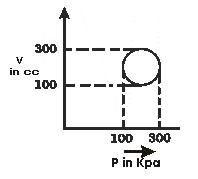Q.3 when water is boiled under a pressure of 2 atm, the heat of vaporization is 2.20 x 106 J Kg-1 and the boiling point is 120 °C. At this pressure 1 Kg of water has a volume 10-3 m3, and 1 kg of steam a volume of 0.824 m3,
(a) Compute the work done when 1 kg of steam is formed at this temperature
(b) Compute the increase in internal energy

Q.4 Figure below shows different paths. connecting state i to state f in P-V diagram along which gas can be taken Rank paths according to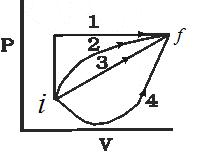(a) Change in internal energy
(b) Work done by gas
(c) The magnitude of energy transferred as heat.

Q.5 Two moles of helium (γ=5/3) are initially at a temperature of 27 °C and occupy a volume of 20L. The helium is first expanded at constant pressure until the volume has tripled, and then adiabatically until the temperature returns to its initial state. given for helium
Cp = 20.78 J/mol-k
(a) Draw diagram of process is P-V plane
(b) What is the total heat supplied in the process
(c) What is the total change in internal energy of helium.
(d) What is the total work done by the helium.
(e) What is the final volume and pressure.

Q.6 Temperature of a system containing 1 mole ideal gas increases by on amount ΔT = 30 K as a result of heating at constant pressure. If gas obtains an amount of heat Q= 3.2 KJ. then calculate
(a) work done by the gas
(b) change in its internal energy
(c) value of γ= Cp / Cv

Q.7 Two moles of a certain ideal gas at a temperature T0 = 300K were cooled isochorically so that the gas pressure reduced two times. Then as a result of the isobaric process the gas expanded till its temperature get back to the initial value.
(a) What would be total change in internal energy.
(b) Total work done by the system.
(c) Find the total amount of heat absorbed by gas in this process

Q.8 An ideal gas where adiabatic exponent is equal to γ, is expanded so that the amount of heat transferred to the gas is equal to the decrease of internal energy find
(a) molor heat capacity of gas in this process
(b) equation of process in variables T, V
(c) Work performed by one mole of gas when its volume increases by η time if T0 is the initial temperature of the gas.

Q.9 An ideal gas is taken from an initial state i to a final state f in such a way that the ratio of the pressure to the absolute temperature remains constant. What will be the work done by the gas.

Q.10 Consider the cyclic process ABCA, shown in the Figure, performed on a sample of 2.0 mole of an ideal gas. A total of 1200 J of heat is withdrawn from the sample as the process. Find the work done by the gas during the part BC.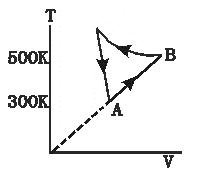Q.11 Consider a cylindrical tube of volume V with adiabatic walls containing an ideal gas. The internal energy of this ideal gas is given by 1.5 μRT. The tube is divided into two equal parts by a fixed diathenic wall. Initially, the pressure and the temperature are P1, T1 on the left side and P2, T2 right. The system is left for sufficient time so that the temperature becomes equal on the two sides.
(a) How much work has been done on the left part.
(b) Find the final temperature on the two sides.
(c) Find the final pressure on the two sides.

Q.12 1 moles of an ideal gas whose adiabatic exponent equals y undergoes a process in which gas pressure relates to the temperature as
P=aT2 where a is constant.
If the temperature get an increment of ΔT,find following
a. Change in Internal energy
b. Work performed by the gas
c. Molar heat capacity of the gas in the process
d. find the amount heat supplied

Q.13 Two vessels A & B of equal volumes V0 are connected by the narrow tube which can be closed by the valve. The vessels are fitted with the piston which can be moved to change the volumes.Intially the valve is open and vessel contains ideal gas whose adiabatic exponents is Y at atmosphere pressure P0 and atmosphere temperature T0.The walls of the vessel A are adiabatic while the walls of the vessels B are diathermic. The valve is now closed and piston is slowly moved to triple the original volume.
a. Find the temperature and pressure in the two vessels.
b The valve is now open for sufficient time so that gases acquire a common temperature and pressure. Find the new values of pressure and temperature

Q.14 1 mole of an ideal gas whose adiabatic exponents is Y is enclosed in the vertical adiabatic vessel fitted with moving frictionless piston whose weight is W and cross-sectional area is A.The atmospheric pressure is P0.Intial volume is V0.Heat is supplied to the gas to double its volume.
a. Find the initial and final state of the gas
b. Find the work done by the gas
C. Find the change in internal energy

Q.15 Two samples A and B whose adiabatic exponents is Y are initially kept in the same state (P0,V0,T0).Now sample A is expanded such that volume becomes 2V0 and Q=0 for the process. Sample B is expanded such that volume becomes 2V0 and ΔU=0 for the process
a. Find the ratio of the final pressure of the two samples
b. Find the ratio of the final temperature of the two samples
c. Find the ratio of the work done by the two samples

Q.16 One mole of an ideal monoatomic gas is taken round the cyclic process ABCDA as shown in figure.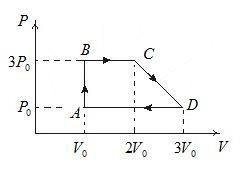a. Work done by the gas
b Heat absorbed by the gas in AB and BC
c. Heat in process CD
d. Find the temperature at C and D
e. Maximum temperature attained by the gas during the cycle
f. Net change in the internal energy and the heat

Q.17 An ice cube of mass .1kg at 0 °C is placed in an isolated container which is at 227° C. The specific heat of the container varies with temperature according to the empirical formula
S=A + BT
where A is 100cal/kg-K and B is 2x10-2 cal/kg-K2
if the final temperature of the container is 27°C.Determine the mass of the container.
Specific heat of water is 103 cal/kg-K
Latent heat of fusion of water is 8x104 cal/kg

Q.18 A gaseous mixture is such that
 Gas No of moles Adiabatic coefficient A 1 1.67 B 2 1.4

The gaseous mixture is taken through an adiabatic process where temperature of the mixture drops by ΔT.
a. Find the work done by the mixture
b. If m1 and m2 are the relative molecular mass of the gas A and B respectively. Find the speed of sound in the mixture at Temperature T0

Q.19 A diatomic gas whose adiabatic coefficient is y=1.4 is taken through XYZAX cycle.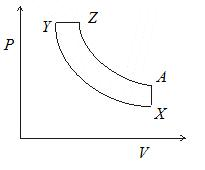Y->Z Isobaric expansion
A->X Isochoric process
find the following
1. Molar heat capacities in each process
2. if the volume ratio is VX/VY=16 and VZ/VY=2 and TY=636° C
a. find the heat absorbed in the process Y->Z
b. find the net work done by the gas in the cycle
c. find the ratio of pressure PY/PX and PZ/PA

Solutions